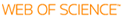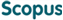BROWSE

Related ResearcherJang, Bongsoo
Computational Mathematical Science Lab (CMS Lab)
Research Interests
• Numerical analysis, dynamics, fractional systems, data sciences

A semi-analytic method with an effect of memory for solving fractional differential equations

Cited 0 times inCited 0 times inTitle
A semi-analytic method with an effect of memory for solving fractional differential equations
Author
Issue Date
2013-12
Publisher
SPRINGER INTERNATIONAL PUBLISHING AG
Citation
ADVANCES IN DIFFERENCE EQUATIONS, v.2013, pp.1 - 14
Abstract
In this paper, we propose a new modification of the multistage generalized differential transform method (MsGDTM) for solving fractional differential equations. In MsGDTM, it is the key how to impose an initial condition in each sub-domain to obtain an accurate approximate solution. In several literature works (Odibat et al. in Comput. Math. Appl. 59:1462-1472, 2010; Alomari in Comput. Math. Appl. 61:2528-2534, 2011; Gokdo&#287;an et al. in Math. Comput. Model. 54:2132-2138, 2011), authors have updated an initial condition in each sub-domain by using the approximate solution in the previous sub-domain. However, we point out that this approach is hard to apply an effect of memory which is the basic property of fractional differential equations. Here we provide a new algorithm to impose the initial conditions by using the integral operator that enhances accuracy. Several illustrative examples are demonstrated, and it is shown that the proposed technique is robust and accurate for solving fractional differential equations.
URI
https://scholarworks.unist.ac.kr/handle/201301/2844
URL
http://www.scopus.com/inward/record.url?partnerID=HzOxMe3b&scp=84897906779
DOI
10.1186/1687-1847-2013-371
ISSN
1687-1839
Appears in Collections:
SNS_Journal Papers
Files in This Item: